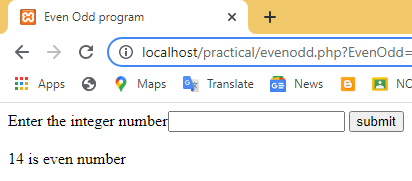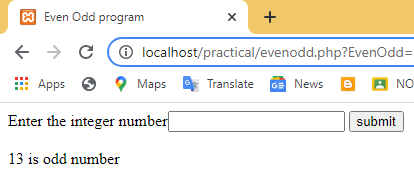# Even Odd program in PHP

In this program, you will find whether numbers entered by users are even or odd.
Even numbers are integers that are exactly divisible by 2 and have no remainder. for Example 2, 10. -16. are even number

Odd numbers are integers that are not divisible by 2. for example 1,3,5,7,.. etc are odd numbers.

## example 1: Even Odd program in PHP

``````<?php
\$n=122;
if(\$n%2==0)
{
echo "\$n is even number";
}
else{
echo"\$n is odd number";
}
?>``````

## output

`122 is even number`

## example 2: Even Odd program using HTML form in PHP

``````<!DOCTYPE html>
<html lang="en">
<title>Even Odd program</title>
<body>
<form>
Enter the integer number<input type="number" name="EvenOdd">
<input type="submit" value="submit" name="click"></br></br>
</form>
</body>
</html>
<?php
if(isset(\$_GET["click"]))
{
\$n=\$_GET['EvenOdd'];
if(\$n%2==0)
{
echo "\$n is even number";
}
else{
echo"\$n is odd number";
}
}
?>``````

## output